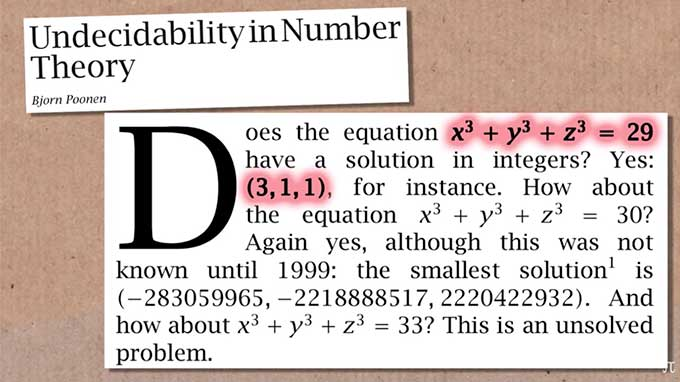## A mathematician found a solution to the much-studied problem 33 = x³ + y³ + z³Solving 29 = x³+ y³+ z³ is easy (29 = 3³ + 1³ + 1³). Solving 33 = x³ + y³ + z³ has confounded number theorists for a very long time. As far back as 1955, they've been using computers to grind away at a solution. This week, University of Bristol mathematician Andrew Booker's computer program solved it after three weeks of number crunching. The answer: (8,866,128,975,287,528)³ + (–8,778,405,442,862,239)³ + (–2,736,111,468,807,040)³ = 33.

From Quanta Magazine:

Until Booker found his solution, it was one of only two integers left below 100 (excluding the ones for which solutions definitely don’t exist) that still couldn’t be expressed as a sum of three cubes. With 33 out of the way, the only one left is 42.

The reason it took so long to find a solution for 33 is that searching far enough up the number line — all the way to 1016, or ten quadrillion, and just as far down into the negative integers — for the right numerical trio was computationally impractical until Booker devised his algorithm. “He has not just run this thing on a bigger computer compared to the computers 10 years ago — he has found a genuinely more efficient way of locating the solutions,” said Tim Browning, a number theorist at the Institute of Science and Technology Austria.